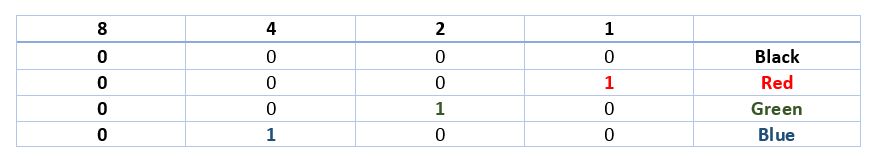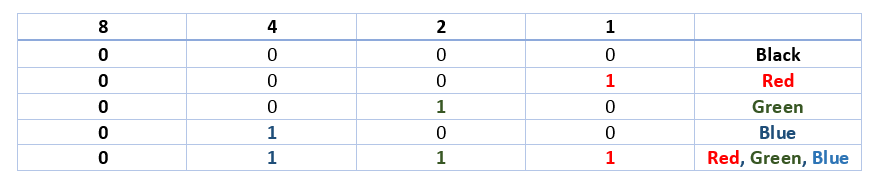# Using bit fields in C#

## Posted by Filip Ekberg on 14 May 2013

Recently I came across a problem where I wanted to allow combinations of a certain criteria so I immediately thought of bit bit fields. This lead me to an interesting answer on StackOverflow for a question on how to use the `FlagsAttribute` with `Enums`.

What I mean about combinations of certain criteria is that let us say that we have a set of colors and I'd like to define that my pants have more than one color. Then somewhere in my application I'd like to check whether or not the pants had a certain color or not. This could of course be solved in many different ways. One other way could be to just store a collection of colors on the pants class. That would however make this article less fun. So let's take a look at how to use bit flags. Both the answer on SO and the link to `FlagsAttribute` above which has some examples use colors for their demonstrations, this is because it's a very common and easy scenario.

Let's say that we define RGB, Red, Green and Blue by using bits. This could mean that we have three colors with bit representations as followed:Now let's say that we have all colors together, that means that we have the following bits:Red, Green and Blue added together! So why does this matter? If you don't know your bits and bytes it's going to be quite difficult to understand. There's an older article that I've written about bits and bytes, check that out if this is all Greek to you!

As you see it just "added" the bits together, but how do we do this in C# then? It looks "so easy" on paper! First we need to fine an enumerator for this.

``````[Flags]
enum Colors {
Black = 0,
Red = 1,
Green = 2,
Blue = 4
}
``````

If you've peeked at the StackOverflow answer linked above, you might already know that using the attribute Flags doesn't do anything at all. Except it's handy if we use reflection and it changes the output when we print the value.

Now, how do we use this enum? It's also easier than you might think!

By using something called "OR" we can add values together and get a nice bit representation. OR is written with a single `|` like this:

``````Colors color = Colors.Red | Colors.Green | Colors.Blue;
``````

The value of `color` will now be 7. Why? If you add the numbers together in the figure which shows the bit representation of Red + Green + Blue, you'll see that you will get the value 7!

If we go back to the original "Problem" now, how do we check if a certain color exists in this bit representation of colors? Let's talk a bit about OR first. What OR does is that it checks two bit representations and once a 1 occurs, a 1 is set in the result. That might sound confusing. So let's say that we have `0001 | 0010` the result of this would be `0011`. So the 1 is dominating here, if there's a 1 in either of the two bit representations that you are "OR"-ing then there's going to be a 1 at that bit in the result.

As there's something called OR, there's most likely something called AND, right? There sure is! That however works a bit different it checks if there's a 1 in both representations. AND is written with a single `&` and you use it pretty much like OR. So if we use AND on the following `0001 | 0011` the result would be `0001` because the first bit is the only one that has a 1 in both the representations.

Now you might have already jumped the gun and figured out how to find out if one color occurs in the combination of colors however I'll just expect that you haven't! It's quite easy though when you think about it for a while. If you can use AND to "filter" out everything that is not exactly as the representation that you want, then you can probably use this to check if there's an occurrence of our bits!

So let us say that we have all the colors `0111` now if we AND this with the color red, which is represented with `0001` the result of this AND operation will be `0001` and thus we found the color red! In C# this would look like this:

``````var hasRedColor = (color & Colors.Red) == Colors.Red;
``````

As of .NET 4 you can also use `Enum.HasFlag` which work like this:

``````if (color.HasFlag(Colors.Blue))
{
Console.WriteLine("Oh Hai there Blue!");
}
``````

Here's a more complete example of how you can play around with this:

``````class Program
{
[Flags]
enum Colors
{
Black = 0,
Red = 1,
Green = 2,
Blue = 4
}
static void Main(string[] args)
{
Colors color = Colors.Red | Colors.Green | Colors.Blue;

if ((color & Colors.Red) == Colors.Red)
{
Console.WriteLine("Oh hai Red");
}
if ((color & Colors.Green) == Colors.Green)
{
Console.WriteLine("Oh hai Green");
}
if ((color & Colors.Blue) == Colors.Blue)
{
Console.WriteLine("Oh hai Blue");
}

Console.WriteLine((byte)Colors.Black);
Console.WriteLine((byte)Colors.Red);
Console.WriteLine((byte)Colors.Green);
Console.WriteLine((byte)Colors.Blue);
Console.WriteLine((byte)color);
Console.WriteLine(color);
}
}
``````

Will you be using this in your applications or do you think this is the completely wrong approach?